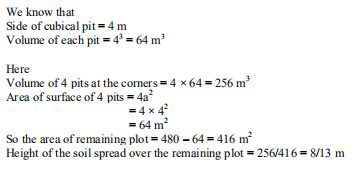Ml aggarwal solutionsOur top 5% students will be awarded a special scholarship to Lido.
Set your child up for success with Lido, book a class today!

A rectangular plot is 24 m long and 20 m wide. A cubical pit of edge 4 m is dug at each of the four

corners of the field and the soil removed is evenly spread over the remaining part of the plot. By what

height does the remaining plot get raised?

• Solution

• Transcript

It is given that

Length of plot (l) = 24 m

Width of plot (b) = 20 m

So the area of plot = l × b = 24 × 20 = 480 \mathrm{m}^{2}"hello and welcome my idea students i am rishabh the math tutor on lido and i'm here a new question it is a rectangular plot is 24 meter long and 20 meter wide a cubical bit of edge four meters stuck at each of the four corners the field and the soil is removed evenly spread over the remaining part of the plot by what height does the remaining plot get raised so without wasting much time let's have a look at the solution so we have been given length of the plot i'm writing l for that is 24 meters whereas the width of the plot i'm writing b for that is 20 meter so the area of the plot is l into b which is 24 into 20 which is equal to 480 meter square now we know that the side of the cubicle bit is 4 meter so side of cubicle bit is 4 meter therefore the volume of each bit is a cube and the side is 4 is nothing but 64 meter cube so therefore the volume of four such bits at the corner is nothing but 4 into 64 which is equal to 256 meter cube also the area of surface of pits is 4 into side square be careful that it is area of 4 surface of 4 pits the areas of 4 pets be careful about it so that means 4 into 4 square which is again nothing but 64 meter square now this means that the remaining area of the plot is equals to 480 minus 64 which is nothing but equals to 416 meter square therefore the height i'm writing height only so height of the soil spread over the remaining plot is nothing but equals to 256 divided by 416 that is the remaining area which is nothing but 8 by 13 meters so the required height is nothing but the 8 by 13 meters so i hope my students got this question well do not forget to hit the subscribe button you can also share your doubts in the comment section see you next time bye bye"

Our top 5% students will be awarded a special scholarship to Lido.Lido

Courses

Teachers

Book a Demo with us

Syllabus

Science
English
Coding

Terms & Policies

NCERT Question Bank

Maths
Science

Selina Question Bank

Maths
Physics
Biology

Allied Question Bank

Chemistry